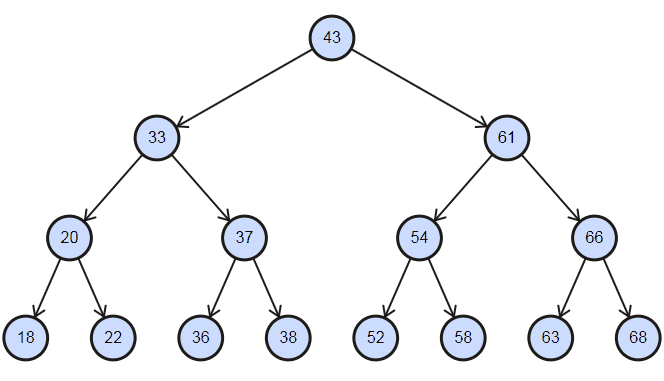#DAY 17
3
Software Development

## [Day 17] 從LeetCode學演算法 - 0098. Validate Binary Search Tree (Medium)

(Binary Search Tree)

``````Question:
Given a binary tree, determine if it is a valid binary search tree (BST).
Assume a BST is defined as follows:
The left subtree of a node contains only nodes with keys less than the node’s key.
The right subtree of a node contains only nodes with keys greater than the node’s key.
Both the left and right subtrees must also be binary search trees.
``````
``````Example 1:
2
/ \
1   3
Input: [2,1,3]
Output: true
``````
``````Example 2:
5
/ \
1   4
/ \
3   6
Input: [5,1,4,null,null,3,6]
Output: false
Explanation: The root node's value is 5 but its right child's value is 4.
``````

1. 任一個節點的左子樹的所有節點值小於該節點自身的值
2. 任一個節點的右子樹的所有節點值大於該節點自身的值
3. 二元搜尋樹的左子樹右子樹也都是二元搜尋樹

(節點分布的很均勻時)，會相當有利於搜尋值，

(記得我們提過二元樹不一定非得要全部都有連接節點，(Binary Serach Tree (Balanced, Complete, Perfect))

In-order的順序為左-中-右，代表每次我們目標要先拿到左邊的值，

[18, 20, 22, 33, 36, 37, 38, 43, 52, 54, 58, 61, 63, 66, 68]的話，

``````inorder(root) {
if root == NIL return
inorder(root.left)
print(root.val)
inorder(root.right)
}
``````

``````let last = NIL
isValidBST(root) {
if root == NIL return true
if !isValidBST(root.left) return false
if last != NIL and root.val <= last.val return false
last = root // 更新上一個節點的位置
return isValidBST(root.right)
}
``````

Java的寫法部分，我們可以宣告一個class的變數last，

Java:

``````/**
* Definition for a binary tree node.
* public class TreeNode {
*     int val;
*     TreeNode left;
*     TreeNode right;
*     TreeNode(int x) { val = x; }
* }
*/
class Solution {
TreeNode last;
public boolean isValidBST(TreeNode root) {
if (root == null) return true;
if (!isValidBST(root.left)) return false;
if (last != null && root.val <= last.val) return false;
last = root;
return isValidBST(root.right);
}
}
``````

Python的部分，請留意到記得在呼叫時使用self的關鍵字，

Python:

``````# Definition for a binary tree node.
# class TreeNode:
#     def __init__(self, x):
#         self.val = x
#         self.left = None
#         self.right = None

class Solution:
def __init__(self):
self.last = None
def isValidBST(self, root: TreeNode) -> bool:
if not root: return True
if not self.isValidBST(root.left): return False
if self.last and root.val <= self.last.val: return False
self.last = root
return self.isValidBST(root.right)
``````

「時間/空間複雜度？」
（O(N), O(1)，因為要走過整個BST，

「可否不用遞迴呢？」
(可以，使用Stack，可以用迴圈讓每次先將root塞入，一路向左邊走將每個左節點放入Stack中，接下來同樣每次取出一個節點，判斷和前一個的大小比較。最後每個左側處理完以後，再將位置移向右節點，迴圈重複即可。)

「可以不用class的變數來解此題嗎？」
(可以，有一種解法是記錄對某個節點的最小值最大值限制，

Java的解法這邊收錄 ryanswizzle在Leetcode的解法如下。)

``````public class Solution {
public boolean isValidBST(TreeNode root) {
return isValid(root, null, null);
}

public boolean isValid(TreeNode root, Integer min, Integer max) {
if(root == null) return true;
if(min != null && root.val <= min) return false;
if(max != null && root.val >= max) return false;

return isValid(root.left, min, root.val) && isValid(root.right, root.val, max);
}
}
``````

0094. Binary Tree Inorder Traversal (Medium)